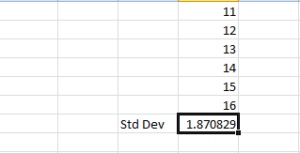### Give us a call

Our team of experts are on hand and ready to help.### Live chat

Ready to chat data? That's what we're here for### Submit an enquiry

Fill out your details and one of the team will be in touch

To calculate the Standard Deviation in Excel, you start by clicking on the formula tab on the ribbon and then click on the More Functions button. From the drop down list choose Statistics and then click on Std Dev button. When the Function Arguments dialogue box opens up, enter the cell range of the list of data that you want to use. Clicking OK will complete the calculation and give you the standard deviation in Excel for the selected list.

Standard deviation in Excel is used to calculate how far each individual data entered on a list deviates from the average of the list as a whole. It is not necessary to calculate the average of a data list to find the standard deviation as the formulas for calculating standard deviation as it is part of the formulas calculation.  This formula has many different uses and using the tools in the formula tab on the ribbon makes it very easy to use.In the above example the numbers 11, to 16 were entered in to cells D1;D6, then selecting the Std Dev formula, we got the answer of approximately 1.87.## Our blog

Get up to speed on what’s happening in the world of Data…